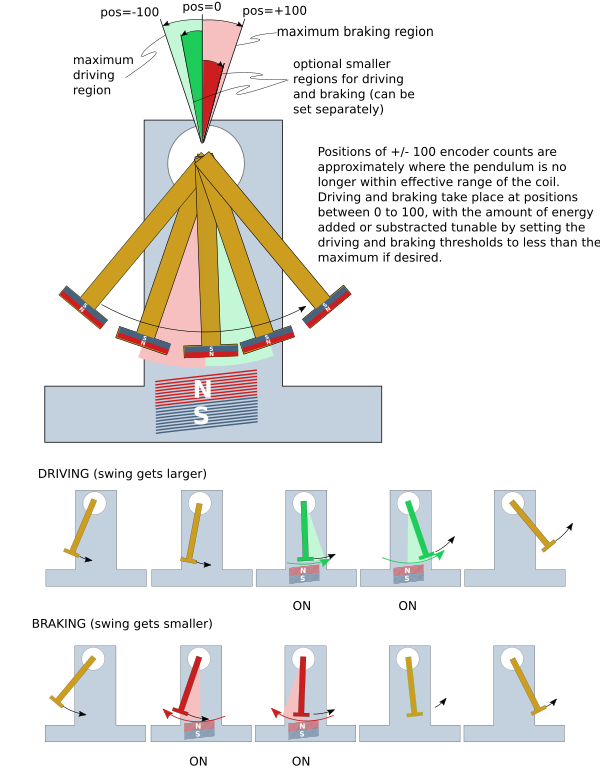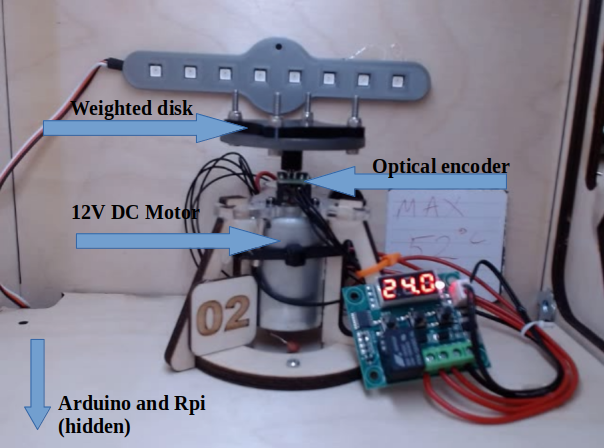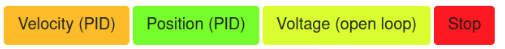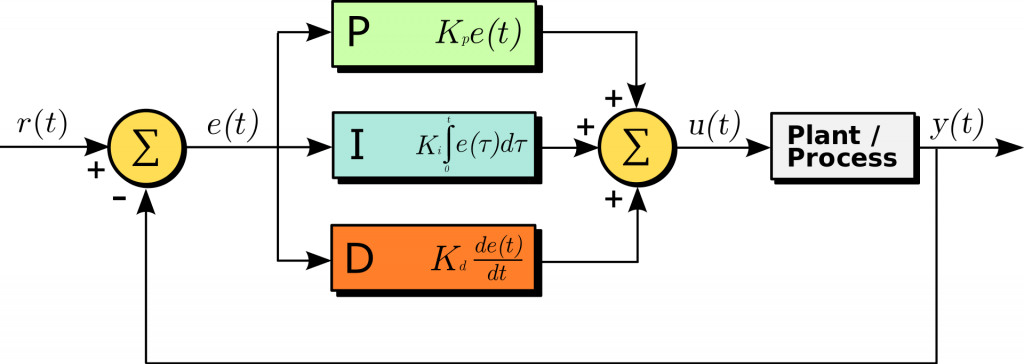Categories

## Exploring the remote labs

On Wednesday 5th May 2021, the University of Edinburgh is hosting an event for prospective undergraduates to give them a taste of practical lab work here in the School of Engineering. Under current travel restrictions, the students are unable to physically be on campus; however, the new remote lab system, designed and built in-house, will allow students to remotely interact with hardware and gain experience of what future hybrid learning may look like in STEM education. We’re also keen that everyone else who is interested in remote labs can have a go too – so this blog post is both for the students coming to our event, and for anyone who has made their way to this site who wants to try things out themselves. We’ll post a link here when general public access to the lab is available.

Two types of experiment will be available for students to explore: a simple pendulum remote lab and a spinning disk with proportional-integral-derivative (PID) controller (both real and simulated hardware). These remote and simulated labs give students the opportunity to explore these systems without the physical and temporal constraints of a traditional lab environment – in other words, they can do practical work from anywhere, at a time that suits them. The user interfaces are designed to encourage open exploration of the hardware, to provide a range of modes to control the equipment and to provide various methods for collecting and analysing data. Analysis can occur within the web app itself using the ‘Table’ and ‘Graph’ tools, including comparison of data to theoretical models with the ‘Function Plotter’. Data can also be downloaded (as a .csv file) for further exploration outside of the interface.

We’re used to seeing new users enjoy controlling something at a distance, so we know it is fun – the real time connection is important for making that emotional connection. When it comes down to getting actual work done, there is still some work to be done on making them seem a little less, well, … remote! We are actively researching student perceptions of different interaction modes. One such mode is having users make analogue measurements of hardware via UI tools, and this can be seen with the ruler and protractor in the pendulum remote lab. Although accuracy and precision could be viewed as a benefit of remote over traditional (proximal) labs; perhaps it is a disadvantage when the intentions of practical work include student skill development and an understanding of experimental error. This is one of the great strengths of remote labs – each experience can be tailored to give the kind of intended learning outcomes that are most important. This adds to the design burden for the creators, and there is a lot of rich work to be done here. In short, though, all of this will lead to design changes over time which enhance the experience for staff and students alike – meanwhile, we get to enjoy the immediate benefits of convenient and immediate access.

Our event is really just about letting students connect to the experiments and have a look around the interface. We encourage student-led exploration of the remote labs – whatever you want to do, is good. And we also want to show some of the depth that you can get to even with apparently simple experiments. So for anyone wanting to try getting into the details, below you will find some information on the lab hardware and then outlines of a few in-depth activities for the pendulum and spinning disk labs. More information on the remote lab UI and individual UI elements can be accessed at:

https://static.practable.io/info/spinner-1.0/index.html

## How the labs work

#### PendulumAvailable modes in the Pendulum remote lab

The electromagnet (or coil), when energised, repels the magnet affixed to the bottom of the pendulum. When the coil is not energised, it is open-circuited and has no effect on the pendulum. We can selectively energise and de-energise the coil in order to control the swing of the pendulum. Switching the coil on when the pendulum is receding from the centre point has the effect of repelling it and maintaining or increasing the swing height – this is the behaviour in ‘Start’ mode. Switching the coil on when the pendulum is approaching the centre point has the opposite effect, acting as a brake that slows the pendulum – ‘Brake’ mode. Driving or braking only occur within a limited range of positions around the zero point. This range can be controlled with the drive and brake settings on the UI. The timing is illustrated below:How the pendulum is electromagnetically driven and braked.

‘Free’ mode leaves the coil open-circuited and de-energised throughout its motion, therefore the pendulum has no drive, but does have the damping effect of air resistance and friction (whilst in motion). In this mode the pendulum will come to a stop slowly. ‘Load’ mode will de-energise the coil, but also short-circuit it so that a current is induced in the coil as the magnet swings past, generating a field around the coil that opposes the pendulum’s motion. This mode brings the pendulum to a stop faster than ‘Free’ mode, but slower than ‘Brake’.

Large amplitude oscillations may cause the encoder to miss position counts and after a long time the drive may be out of sync with the pendulum motion. The ‘Cal’ (calibrate) mode waits for the pendulum to stop and zeroes the encoder position at the equilibrium point (hanging vertically downwards).

More information on the pendulum experiment, and the firmware that is run on the Arduinos controlling the hardware, can be found at:

https://github.com/timdrysdale/penduino

#### Spinning disk PID controllerOverview of the spinning disk hardware.Modes available in the spinning disk PID controller

The spinning disk remote lab consists of a disk attached to a DC motor with an optical encoder for measuring angular position (and to calculate angular velocity). An Arduino runs the firmware for controlling the motor and a Raspberry Pi is used for streaming video and data.

The spinning disk can be put into 3 different modes: voltage, position (PID) and velocity (PID). Voltage mode has no feedback loop and simply allows a voltage to be applied to the motor (either as a step or ramp input). The other two modes use a proportional, integral, derivative (PID) controller in order to set the motor to a specific position or velocity. The feedback loop is shown in the diagram below. The plant in our case is the motor/disk system. r(t) is the set point (either the position or speed we set our disk to) and y(t) is the measured value (of position or speed). The error, e(t) = r(t) – y(t), is used to calculate a correction based on the PID terms to apply a new voltage to the motor (plant).PID controller feedback loop

More information on PID controllers in general can be found at:

https://en.wikipedia.org/wiki/PID_controller

And code for running our spinning disk PID controller can be found at:

https://github.com/dpreid/pidcontroller

[Note: the README has not been updated to reflect the current state machine in the above repository]

## Pendulum Activities

#### 1. Period vs amplitude

Systems that are governed by simple harmonic motion have a time period that is independent of the amplitude of motion. Is the compound pendulum simple harmonic over all drive strengths?

• Find the smallest drive that gives you oscillatory motion. Measure the time period for this oscillation as accurately as you can.
• Continue increasing the drive by a few percent and measure the period each time.
• Does the period remain constant?
• The period of a simple pendulum is calculated using:

What is the equivalent length of a simple pendulum that would give the same period as this compound pendulum when it is performing simple harmonic motion?

• How does your measured length compare to the actual length of this compound pendulum?
• Use the ‘Trigonometric’ function plotter to model the pendulum oscillation. How does the theoretical time period compare to your calculated time period?

#### 2. Estimate of energy loss per oscillation

The total energy of the pendulum can be measured as its kinetic energy through the equilibrium position (when potential energy is 0). Kinetic energy is calculated as:

Where I is the moment of inertia of the pendulum and ω is its angular velocity. We don’t easily know the moment of inertia of this compound pendulum, but we want to calculate the fractional change in energy over one swing and this information is therefore not necessary.

• Drive the pendulum at around 70%.
• Set the pendulum to ‘Free’ mode.
• Measure the speed of the pendulum through the equilibrium position on a swing and then the next swing.
• What is the fractional change in the kinetic energy through the equilibrium position over one swing?
• Estimate how many swings the pendulum should complete before stopping?
• Count the number of swings the pendulum actually takes to stop and compare to your calculation? Is there a difference? Why?

#### 3. Damping coefficient

When set to ‘Free’ mode the pendulum will swing under the effects of air resistance and mechanical friction. From the moment the pendulum is set to ‘Free’ the amplitude of the oscillation can be modelled using the following equation:

Where A(t) is the amplitude at time, t, A0 is the initial amplitude and b is the damping coefficient.

• Set the drive to 100% and wait until the pendulum reaches a consistent amplitude.
• Start recording data on the graph and press the ‘f’ key at the same time.
• Stop recording once the pendulum reaches a low amplitude oscillation.
• Use the graph (and table if you like) to identify the amplitude and time for each peak of the decay curve. Note these down.
• In spreadsheet software, can you show that the amplitude decays according to the above equation and find a value for the damping coefficient, b. [HINT: taking the natural log of both side of the above equation will give you an expression for ln A against t, how can b be found from a plot of lnA against t?]
• Check your calculated value using the exponential function plot on the graph.

## Spinning disk PID controller (simulated)

#### 1. Step response of motor

Motors can be parametrised by their gain and time constant for a step input of voltage. The gain of a motor can be calculated as the increase in angular velocity per unit volt applied. The time constant is measured as the time taken to reach 63% of its maximum angular velocity.

• Using the orange disk, make sure that ‘Select mode’ is set to ‘Speed open loop’ and that Input mode is set to ‘Step’.
• Set the voltage slider to any value you like and note it down.
• Click ‘Set’ and wait until the graph levels out to a constant speed.
• Calculate the gain of the motor:
• Calculate the time constant as the time taken to reach 63% of the maximum measured speed.
• Select the plot function ‘Step (1st Order)’ and input the values that you calculated – step size is your input voltage, K is gain and τ is time constant. Just leave t0 as 0. Does the theoretical step response (red curve) look similar to your calculated values.
• Repeat for the green and/or blue disks – which values remain constant and which change?

#### 2. Proportional Controller

PID controllers provide feedback control in systems such as automatic speed and steering in cars. They do this by measuring the error in the current output of the system compared to the desired set point and feeding this back to the input of the controller.

• Using any disk, select mode ‘Position PID’ and Input mode ‘Step’.
• Leave the PID Parameters at the default values (Kp = 1, Ki = 0, Kd = 0).
• Set the step size to 1 rad and click ‘Set’.
• Wait until the graph stops plotting or the oscillations have reached a small value and then click ‘Stop’.
• Describe the behaviour of a proportional controller.
• How long did the disk take to reach the first maximum? What angle was the first maximum? Note these down.
• Increase Kp a few times and make the same measurements as above.
• What happens to the time to first maximum and the maximum angle as Kp increases?

Extension: What effect does changing the Ki and Kd parameters have on the response?